# [翻译] 神经网络与深度学习 第三章 提升神经网络学习的效果 - Chapter 3 Improving the way neural networks learn

> 第三章 提升神经网络学习的效果

# 提升神经网络学习的效果

## 交叉熵代价函数$C(w, b) \equiv \frac 1{2n} \sum_x ||y(x) - a||^2 \tag{6}$

$C = \frac {(y - a)^2}{2}, \tag{54}$

\begin{aligned} \frac {\partial C}{\partial w} &= (a - y) \sigma' (z) x = a\sigma' (z) \\ \frac {\partial C}{\partial b} &= (a - y) \sigma' (z) = a \sigma' (z), \tag{55, 56} \end{aligned}### 介绍交叉熵代价函数$C = - \frac 1{n} \sum_x [y \ln a + (1-y) \ln (1-a) ], \tag{57}$

*为了证明这一点，我将假设所有的期望输出 $y$ 均为 $0$$1$。在解决分类问题的时候，这是一种常见的情况，例如在计算布尔函数的时候。如果我们不做这个假设，该如何理解呢？详见本节最后的习题。

\begin{aligned} \frac {\partial C}{\partial w_j} &= - \frac 1{n} \sum_x \left (\frac {y}{\sigma (z)} - \frac {(1-y)}{1-\sigma (z)} \right ) \frac {\partial \sigma}{\partial w_j} \\ &= - \frac 1{n} \sum_x \left ( \frac y{\sigma (z)} - \frac {(1-y)}{1-\sigma (z)} \right ) \sigma' (z) x_j. \end{aligned} \tag {58, 59}

$\frac {\partial C}{\partial w_j} = \frac 1{n} \sum_x \frac {\sigma' (z) x_j}{\sigma (z) (1-\sigma (z))} (\sigma (z) - y). \tag {60}$

$\frac {\partial C}{\partial w_j} = \frac 1{n} \sum_x x_j (\sigma (z) - y). \tag {61}$

$\frac {\partial C}{\partial b} = \frac 1{n} \sum_x (\sigma (z) - y). \tag {62}$

#### 习题

• 验证 $\sigma' (z) = \sigma (z) (1 - \sigma (z))$$C = - \frac 1{n} \sum_x \sum_j \left [ y_j \ln a_j^L + (1 - y_j) \ln (1 - a_j^L) \right ]. \tag {63}$

*当然，在我们的网络中并没有概率元素，所以它们并不是真的概率。

#### 习题

• 一个人也许在刚接触交叉熵的时候会感到很困难，因为记住 $y$$a$ 的各种位置并不容易，$- [y \ln a + (1-y) \ln (1-a)]$$-[a \ln y + (1-a) \ln (1-y)]$ 哪个是正确的？当 $y = 0$$1$ 的时候，上面第二个表达式会发生什么？这个问题会与第一个表达式冲突吗？请解释为什么是，或者为什么不是。
• 在本章的开头，我们对单个神经元进行了讨论，我说对于所有的训练输入，当 $\sigma (z) \approx y$ 的时候，交叉熵的值会很小。这个陈述在 $y = 0$$1$ 的时候成立。在分类问题中，$y$ 通常符合这个要求，但在其他问题（例如回归问题，regression problems）中，$y$ 的值有时候会处于 $0$$1$ 之间。请证明此时交叉熵的值对于所有训练输入当 $\sigma (z) = y$ 的时候，仍旧是最小化的。在本例中交叉熵拥有如下值：
$C = - \frac 1{n} \sum_x \left [ y \ln y + (1 - y) \ln (1 - y) \right ]. \tag {64}$
其中分量 $-[y\ln y + (1-y) \ln (1-y)]$ 有时候又被称为二元熵binary entropy）（译者注：中文翻译不很统一，敬请谅解。）。

#### 难题

• 多层多神经元网络
如上章所介绍的，输出层中，权值对于二次代价的偏导为：
$\frac {\partial C}{\partial w_{jk}^L} = \frac 1{n} \sum_x a_k^{L-1} (a_j^L - y_j) \sigma' (z_j^L). \tag {65}$
当输出神经元在错误的值附近饱和的话，$\sigma' (z_j^L)$ 项将会造成学习缓慢。证明对于交叉熵代价，对于单个训练样本 $x$ 的输出误差 $\delta^L$
$\delta^L = a^L - y. \tag {66}$
用这个表达式证明，输出层代价对于权值的偏导为：
$\frac {\partial C}{\partial w_{jk}^L} = \frac 1{n} \sum_x a_k^{L-1} (a_j^L - y_j). \tag {67}$
$\sigma' (z_j^L)$ 项被抵消了，所以交叉熵避免了学习缓慢的问题。结论对于biases也适用。如果你想证明一下，o(￣ヘ￣o＃)，请便！
• 当我们在输出层使用线性神经元的时候，应用二次代价函数
设想我们有一个多层多神经元网络，其中输出层的所有神经元都是线性神经元linear neurons），即神经元没有sigmoid激活函数，其输出是简单的 $a_j^L = z_j^L$。证明当我们使用二次代价函数的时候，对于每一个训练样本 $x$，其输出误差 $\delta^L$
$\delta^L = a^L - y \tag {68}$
与之前的问题相似，用这个表达式去证明，输出层中代价对于权值和biases的偏导分别为
\begin{aligned} \frac {\partial C}{\partial w_{jk}^L} &= \frac 1{n} \sum_x a_k^{L-1} (a_j^L - y_j) \\ \frac {\partial C}{\partial b_j^L} &= \frac 1{n} \sum_x (a_j^L - y_j). \end{aligned} \tag {69, 70}
这证明了当输出层的神经元为线性神经元的时候，二次代价函数并不会引起任何学习缓慢的问题。所以在这种情况下，二次代价函数，是一种合适的代价函数。

### 使用交叉熵去分类MNIST数字

*在第一章中，我们使用二次代价函数，学习率设置为 $\mu = 3.0$。正如前面所讨论的，如果代价函数发生了改变，去准确地说出是否使用“相同”的学习率是不可能的。对于不同的代价函数，应该用实验的方法去寻找最佳的学习率。

>>> import mnist_loader
>>> training_data, validation_data, test_data = \
>>> import network2
>>> net = network2.Network([784, 30, 10], cost=network2.CrossEntropyCost)
>>> net.large_weight_initializer()
>>> net.SGD(training_data, 30, 10, 0.5, evaluation_data=test_data,
... monitor_evaluation_accuracy=True)


### 交叉熵意味着什么？它从何而来？

\begin{aligned} \frac {\partial C}{\partial w_j} &= x_j (a - y) \\ \frac {\partial C}{\partial b} &= (a - y) \end{aligned} \tag {71, 72}

$\frac {\partial C}{\partial b} = \frac {\partial C}{\partial a} \sigma' (z). \tag {73}$
$\sigma' (z) = \sigma (z) (1 - \sigma (z)) = a (1 - a)$ 带入公式，得到：
$\frac {\partial C}{\partial b} = \frac {\partial C}{\partial a} a (1 - a). \tag {74}$

$\frac {\partial C}{\partial a} = \frac {a - y}{a (1 - a)}. \tag {75}$

$C = - [y \ln a + (1 - y) \ln (1 - a) ] + \text {constant}, \tag {76}$

$C = - \frac 1{n} \sum_x [y \ln a + (1 - y) \ln (1 - a) ] + \text {constant}, \tag {77}$

#### 难题

• 我们已经讨论了很多当使用二次代价函数，神经元饱和的时候可能导致的学习缓慢的问题。还有另外一个阻碍学习的因素，即公式(61)中的 $x_j$ 项，当输入 $x_j$ 趋向于零的时候，相应的权值 $w_j$ 会更新的很慢。请解释，为什么不能通过更换代价函数的方式，去消掉 $x_j$ 项。

### Softmax

Softmax的想法是为我们的神经网络定义一种新的输出层。与sigmoid层相似，它从构造加权输入* $z_j^L = \sum_k w_{jk}^L a_k^{L-1} + b_j^L$ 开始。当然，我们并不会使用sigmoid函数来获得输出。相反，在softmax层中，我们用Softmax函数去处理 $z_j^L$。根据这个函数，第 $j$ 个神经元的激活 $a_j^L$ 为：
$a_j^L = \frac {e^{z_j^L}}{\sum_k e^{z_k^L}}, \tag {78}$

*在描述softmax的时候，我们会频繁地用到上一章中介绍的数学符号。如果你有点忘记的话，可以去复习一下。

（译者注：下面是我录制的Gif图片！CSDN不支持JavaScript！想看原网页的！请点击后面的链接！Xovee非常帅！$\sum_j a_j^L = \frac {\sum_j e^{z_j^L}}{\sum_k e^{z_k^L}} = 1. \tag {79}$

#### 习题

• 构建一个简单的模型，证明网络使用sigmoid输出层的话，其输出激活 $a_j^L$ 的和并不总等于 $1$

#### 习题

• Softmax的单调性
证明 $\partial a_j^L / \partial z_k^L$$j = k$ 的情况下为正数，$j \ne k$ 的情况下为负数。从而保证增加 $z_j^L$ 可以增加 $a_j^L$，并且使其他的输出激活减少。我们在滑动条那里经过了一些实验，但这是一个严谨的证明。
• Softmax的非局部性Non-locality
sigmoid层的一个优点是，输出 $a_j^L$ 是对应的加权输入的函数，即 $a_j^L = \sigma (z_j^L)$。请解释为什么在softmax层中：任何一个输出激活 $a_j^L$ 取决于所有的加权输出。

#### 难题

• 反转Softmax层
设想我们的网络拥有一个softmax输出层，激活 $a_j^L$ 已知，证明其对应的加权输入拥有如下的形式 $z_j^L = \ln a_j^L + C$，其中的常数 $C$ 独立于 $j$

$C \equiv -\ln a_y^L. \tag {80}$

\begin{aligned} \frac {\partial C}{\partial b_j^L} &= a_j^L - y_j \\ \frac {\partial C}{\partial w_{jk}^L} &= a_k^{L-1} (a_j^L - y_j) \end{aligned} \tag {81, 82}

*在这里，$y$ 与我们之前使用的 $y$ 有一些不同。在上一段我们用 $y$ 来表示网络的期望输出，即对于数字 $7$ 我们实际输出 “$7$”。但是在这里，$y$ 表示输出激活向量，这个向量表示 $7$，即除了第七位为 $1$ 以外，其他位均为 $0$

#### 难题

• 推导公式(81)和公式(82)
• “Softmax”这个名字从何而来？
如果我们改变softmax函数，从而使输出激活为：
$a_j^L = \frac {e^{cz_j^L}}{\sum_k e^{cz_k^L}}, \tag {83}$
其中 $c$ 是一个正数。如果 $c = 1$，该公式即是标准的softmax函数。如果使用不同的 $c$，我们将获得不同的函数。证明在这种情况下，输出激活依旧服从一种概率分布，就像通常的softmax一样。假如我们允许 $c$ 是一个很大的数，也就是说 $c \rarr \infin$。输出激活 $a_j^L$ 的极限值为多少？在解决这几个问题之后，你应该就能理解，为什么在 $c = 1$ 的情况下，该函数被当做是最大化函数的“柔化（softened）”版本。这便是“softmax”这个名字的由来。
• 使用softmax和对数似然代价的反向传播算法
在上一章中我们证明了使用sigmoid层的反向传播算法。为了将其推广到softmax层，我们需要对输出层的误差 $\delta_j^L \equiv \partial C / \partial z_j^L$ 定义一个表达式。证明这个合适的表达式为：
$\delta_j^L = a_j^L - y_j. \tag {84}$
使用这个表达式，我们可以把反向传播算发推广到softmax输出层和对数似然代价。

## 过拟合和正则化

*这个典故来自于Freeman Dyson的一篇有趣的文章，他是flawed model的发明者之一。四个参数大象的相关故事你可以在这里找到。

Fermi和Neumann对拥有四个参数的模型表示怀疑。而我们拥有30个隐藏神经元的神经网络，它有大约24,000个参数！这个数字已经非常大了。如果网络拥有100个隐藏神经元，那它将有大约80,000个参数，对于某些最先进的深度神经网络，这个数字甚至达到了数十亿。我们应该相信这样的结果吗？

>>> import mnist_loader
>>> training_data, validation_data, test_data = \
>>> import network2
>>> net = network2.Network([784, 30, 10], cost=network2.CrossEntropyCost)
>>> net.large_weight_initializer()
>>> net.SGD(training_data[:1000], 400, 10, 0.5, evaluation_data=test_data,
... monitor_evaluation_accuracy=True, monitor_training_cost=True)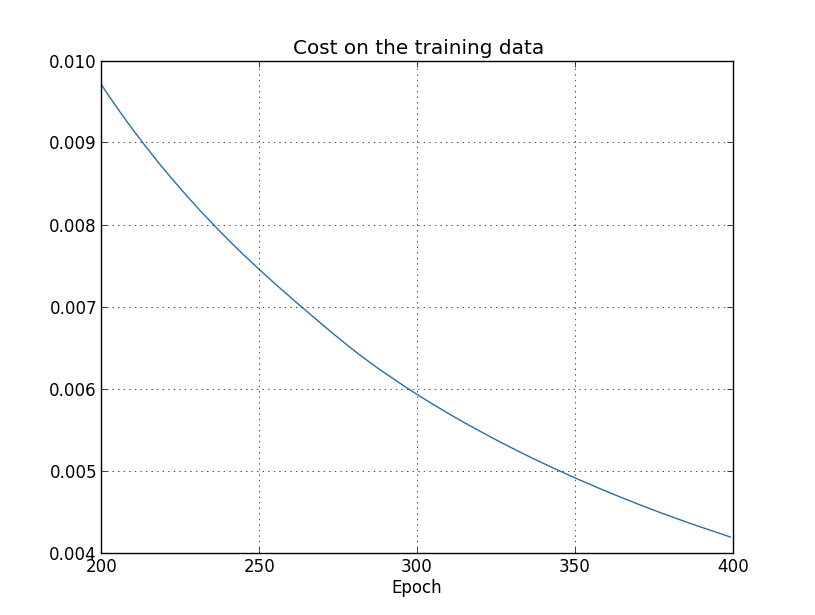*这张图片以及下面的四张图片由overfitting.py程序生成。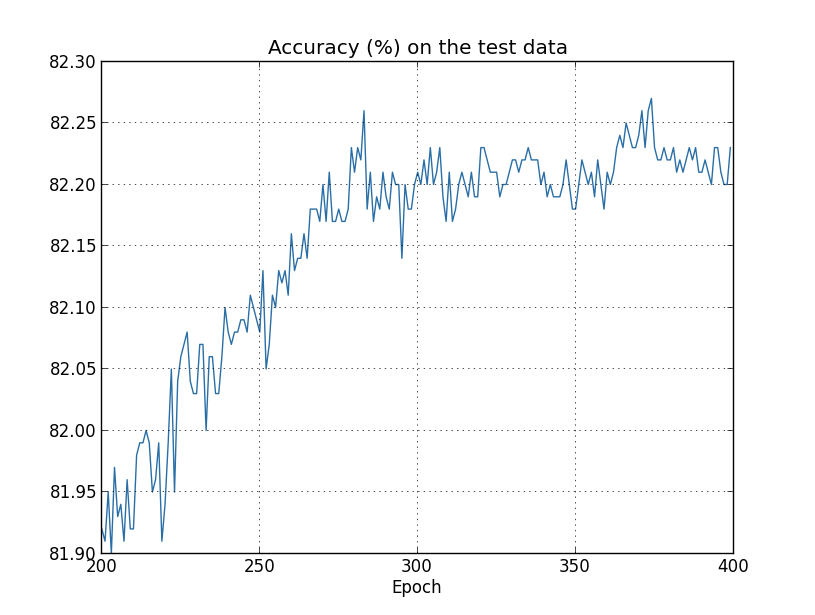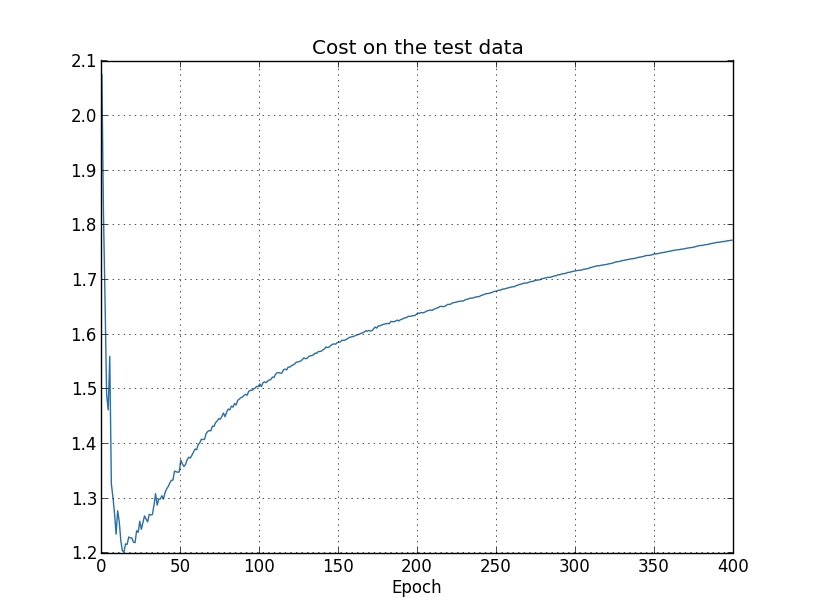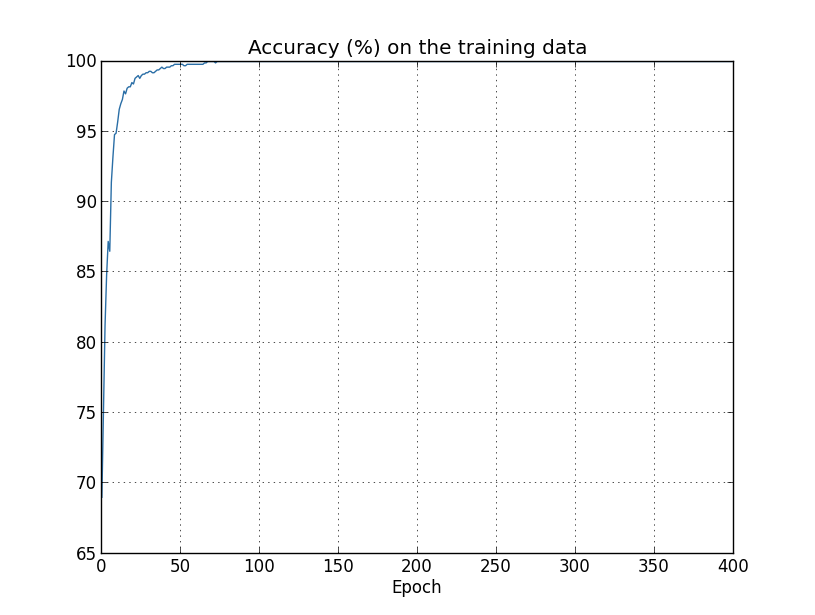>>> import mnist_loader
>>> training_data, validation_data, test_data = \


*这里需要一些判断来确认该何时停止。在前面的图片中，我们判断大约在280轮的时候准确度开始饱和。这似乎太过于悲观了。神经网络在训练中有时候会停滞一段时间，然后继续提升。如果网络在400轮之后可以继续进行学习，我并不会感到惊讶。只不过这种现象发生的概率太小了。我们的早停需要一些或多或少更为激进的策略。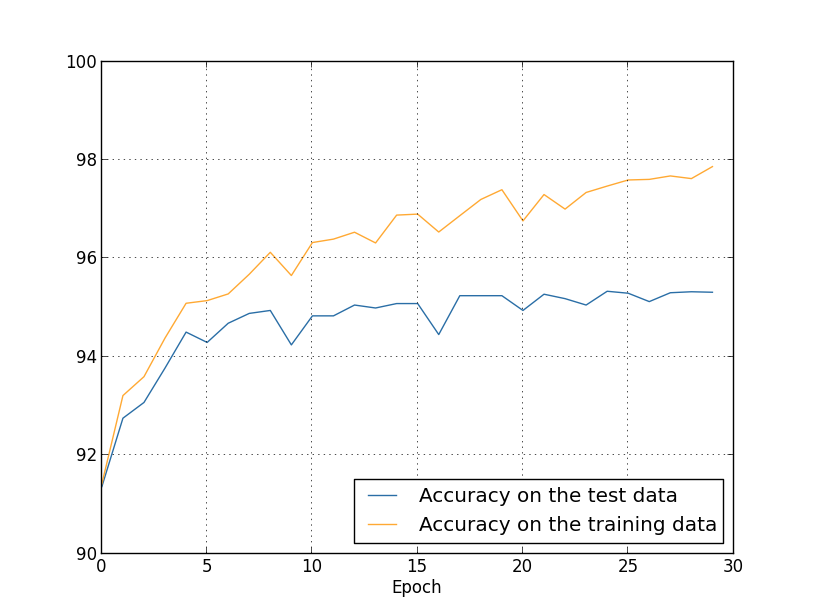### 正则化

$C = - \frac 1{n} \sum_{xj} \left [ y_j \ln a_j^L + (1 - y_j) \ln (1 - a_j^L) \right ] + \frac {\lambda}{2n} \sum_w w^2. \tag {85}$

$C = \frac 1{n} \sum_x ||y - a^L||^2 + \frac {\lambda}{2n} \sum_w w^2. \tag {86}$

$C = C_0 + \frac {\lambda}{2n} \sum_w w^2, \tag {87}$

\begin{aligned} \frac {\partial C}{\partial w} &= \frac {\partial C_0}{\partial w} + \frac {\lambda}{n} w \\ \frac {\partial C}{\partial b} &= \frac {\partial C_0}{\partial b} \end{aligned} \tag {88, 89}

$b \rarr b - \eta \frac {\partial C_0}{\partial b}. \tag {90}$

\begin{aligned} w &\rarr w - \eta \frac {\partial C_0}{\partial w} - \frac {\eta \lambda}{n} w \\ &= \left ( 1 - \frac {\eta \lambda}{n} \right ) w - \eta \frac {\partial C_0}{\partial w}. \end{aligned} \tag {91, 92}

$w \rarr \left (1 - \frac {\eta \lambda}{n} \right ) w - \frac \eta m \sum_x \frac {\partial C_x}{\partial w}, \tag {93}$

*参考公式(20)
$w_k \rarr w_k' = w_k - \frac \mu m \sum_j \frac {\partial C_{X_j}}{\partial w_k} \tag {20}$

$b \rarr b - \frac \eta m \sum_x \frac {\partial C_x}{\partial b}, \tag {94}$

*参考公式(21)
$b_l \rarr b_l' = b_l - \frac \mu m \sum_j \frac {\partial C_{X_j}}{\partial b_l}$

>>> import mnist_loader
>>> training_data, validation_data, test_data = \
>>> import network2
>>> net = network2.Network([784, 30, 10], cost=network2.CrossEntropyCost)
>>> net.large_weight_initializer()
>>> net.SGD(training_data[:1000], 400, 10, 0.5,
... evaluation_data=test_data, lmbda = 0.1,
... monitor_evaluation_cost=True, monitor_evaluation_accuracy=True,
... monitor_training_cost=True, monitor_training_accuracy=True)Okay，让我们开始训练网络，先对权值进行重新初始化：

>>> net.large_weight_initializer()
>>> net.SGD(training_data, 30, 10, 0.5,
... evaluation_data=test_data, lmbda = 5.0,
... monitor_evaluation_accuracy=True, monitor_training_accuracy=True)>>> net = network2.Network([784, 100, 10], cost=network2.CrossEntropyCost)
>>> net.large_weight_initializer()
>>> net.SGD(training_data, 30, 10, 0.5, lmbda=5.0,
... evaluation_data=validation_data,
... monitor_evaluation_accuracy=True)


### 为什么正则化可以帮助减轻过拟合现象？*我没有展示公式的系数，你可以很容易地用例如Numpy的polyfit函数等得到这些系数。如果你好奇的话，你可以在源代码中看到公式的确切形式。它定义在程序的第14行的p(x)函数里。*这个故事来自于历史学家Charles Weiner对物理学家Richard Feynman的一个采访

*这个问题也就是归纳的问题。苏格兰哲学家David Hume在他的一篇广为人知的文章《An Enquiry Concerning Human Understanding》（1748）里对其进行了讨论。David Wolpert和William Macready在1997年提出的“没有免费的午餐理论”给这个归纳问题一个现代的机器学习的形式。

*参考Yann LeCun、Léon Bottou、Yoshua Bengio和Patrick Haffne在1998年发表的论文《In Gradient-Based Learning Applied to Document Recognition》。

### 其他的正则化技巧

L1正则化：它会在未正则化的代价函数之后添加一项所有权值的绝对值的和：
$C = C_0 + \frac \lambda n \sum_w |w|. \tag {95}$

$\frac {\partial C}{\partial w} = \frac {\partial C_0}{\partial w} + \frac \lambda n \text{sgn}(w), \tag {96}$

$w \rarr w' = w - \frac {\eta \lambda}{n} \text {sgn} (w) - \eta \frac {\partial C_0}{\partial w}, \tag {97}$

$w \rarr w' = w \left (1 - \frac {\eta \lambda}{n} \right ) - \eta \frac {\partial C_0}{\partial w}. \tag {98}$

*参考公式(93)：
$w \rarr \left (1 - \frac {\mu \lambda}{n} \right ) w - \frac \mu m \sum_x \frac {\partial C_x}{\partial w}, \tag {93}$

Dropout：Dropout相对于正则化来说非常不同。不像L1和L2正则化，dropout并不对代价函数进行修改，相反，dropout对网络本身进行修改。让我先来描述dropout工作的基本机制，然后介绍其为什么可以减轻过拟合效果。*ImageNet Classification with Deep Convolutional Neural Networks, by Alex Krizhevsky, Ilya Sutskever, and Geoffrey Hinton (2012).

*Improving neural networks by preventing co-adaptation of feature detectors by Geoffrey Hinton, Nitish Srivastava, Alex Krizhevsky, Ilya Sutskever, and Ruslan Salakhutdinov (2012). 这篇论文讨论了很多我在这个简短地介绍里忽略了的细节问题。

*下面的三张图片由程序more_data.py生成*Best Practices for Convolutional Neural Networks Applied to Visual Document Analysis, by Patrice Simard, Dave Steinkraus, and John Platt (2003).

#### 习题

• 就像我们前面讨论过的，一种扩大MNIST数据集的方法是稍微旋转图片。那么，如果我们随意的旋转图片，可能会发生什么问题？*图片是由程序more_data.py生成。

*这里有一个惊人的例子，你可以在这篇Michele Banko和Eric Brill在2001年写的论文《Scaling to very very large corpora for natural language disambiguation》里看到。

#### 难题

• （研究难题） 我们如何去推断机器学习算法在非常大的数据集上的表现呢？（我们暂时还没有这样大的数据集）对于任何一个给定的算法，我们非常自然地想到去定义一个渐进（asymptotic）的表现。一个很快但也很取巧的方法是去拟合图片中的曲线。这种方法的一个反对意见是：不同方法可能拟合出来不同的代表渐进表现的曲线。你能找到一种对于某个特定曲线的公正的拟合原则吗？如果是的话，比较不同机器学习算法的渐进表现。

## 权值初始化*我们在第二章中对此进行了更详细的讨论，即当使用反向传播公式的时候，饱和神经元的权值学习的很慢。#### 习题

• 证明 $z = \sum_j w_j x_j$ 的标准差为 $\sqrt {3/2}$。这也许可以帮助你去理解：（a）独立随机变量的和的方差，是个体随机变量的方差的和；（b）方差是标准差的平方。

>>> import mnist_loader
>>> training_data, validation_data, test_data = \
>>> import network2
>>> net = network2.Network([784, 30, 10], cost=network2.CrossEntropyCost)
>>> net.large_weight_initializer()
>>> net.SGD(training_data, 30, 10, 0.1, lmbda = 5.0,
... evaluation_data=validation_data,
... monitor_evaluation_accuracy=True)


>>> net = network2.Network([784, 30, 10], cost=network2.CrossEntropyCost)
>>> net.SGD(training_data, 30, 10, 0.1, lmbda = 5.0,
... evaluation_data=validation_data,
... monitor_evaluation_accuracy=True)*生成图片的程序是weight_initialization.py*Practical Recommendations for Gradient-Based Training of Deep Architectures, by Yoshua Bengio (2012).

#### 难题

• 将优化的权值初始化方法与正则化建立联系
L2正则化有时候与这个新的权值初始化方法表现的非常相似。设想我们在使用旧的权值初始化方法。这里有一些启发式的讨论：（1）如果 $\lambda$ 不是非常的小，那么第一轮的训练将主要受到权值衰减的影响；（2）如果 $\eta \lambda \ll n$，对于每一个epoch，权值将会受到因子为 $\text {exp}(-\eta \lambda / m)$ 的衰减；（3）如果 $\lambda$ 不是很大的话，当权值大约变小到 $1 / \sqrt {n}$ 的时候，权值衰减效果将会减弱，其中 $n$ 是网络中权值的总数。证明这些条件在本章中的所有例子中都满足。

## 重温手写数字识别：代码

network2.py的核心是Network类，我们用它去表示神经网络。首先用一个表示网络的层的列表sizes初始化一个Network的实例，然后选择将使用的cost类型，默认为交叉熵：

class Network(object):

def __init__(self, sizes, cost=CrossEntropyCost):
self.num_layers = len(sizes)
self.sizes = sizes
self.default_weight_initializer()
self.cost=cost


    def default_weight_initializer(self):
self.biases = [np.random.randn(y, 1) for y in self.sizes[1:]]
self.weights = [np.random.randn(y, x)/np.sqrt(x)
for x, y in zip(self.sizes[:-1], self.sizes[1:])]


    def large_weight_initializer(self):
self.biases = [np.random.randn(y, 1) for y in self.sizes[1:]]
self.weights = [np.random.randn(y, x)
for x, y in zip(self.sizes[:-1], self.sizes[1:])]


large_weight_initializer方法大多数情况下只是用来做比较，我不建议你在实际应用里去使用它。

class CrossEntropyCost(object):

@staticmethod
def fn(a, y):
return np.sum(np.nan_to_num(-y*np.log(a)-(1-y)*np.log(1-a)))

@staticmethod
def delta(z, a, y):
return (a-y)


*如果你还不熟悉Python的静态方法的话，你可以忽略@staticmethod，把fndelta当做普通的方法。如果你对细节感到困惑的话，@staticmethod是告诉Python解释器，它后面的方法并不依赖于其所在的类。这就是为什么self没有给fndelta方法传递参数的原因。

$\delta^L = a^L - y. \tag {99}$

*公式(99)与公式(66)完全相同。

class QuadraticCost(object):

@staticmethod
def fn(a, y):
return 0.5*np.linalg.norm(a-y)**2

@staticmethod
def delta(z, a, y):
return (a-y) * sigmoid_prime(z)


*参考公式(30)
$\delta^L = (a^L - y) \odot \sigma' (z^L) \tag {30}$

"""network2.py
~~~~~~~~~~~~~~

An improved version of network.py, implementing the stochastic
gradient descent learning algorithm for a feedforward neural network.
Improvements include the addition of the cross-entropy cost function,
regularization, and better initialization of network weights.  Note
that I have focused on making the code simple, easily readable, and
easily modifiable.  It is not optimized, and omits many desirable
features.

network.py程序的改进版本，实现了对前馈神经网络的随机梯度下降算法。

"""

#### Libraries 库
# Standard library 标准库
import json
import random
import sys

# Third-party libraries 第三方库
import numpy as np

#### Define the quadratic and cross-entropy cost functions
#### 定义了二次代价函数和交叉熵代价函数

@staticmethod
def fn(a, y):
"""Return the cost associated with an output a and desired output y.
"""
return 0.5*np.linalg.norm(a-y)**2

@staticmethod
def delta(z, a, y):
"""Return the error delta from the output layer. """
return (a-y) * sigmoid_prime(z)

class CrossEntropyCost(object):

@staticmethod
def fn(a, y):
"""Return the cost associated with an output a and desired output
y.  Note that np.nan_to_num is used to ensure numerical
stability.  In particular, if both a and y have a 1.0
in the same slot, then the expression (1-y)*np.log(1-a)
returns nan.  The np.nan_to_num ensures that that is converted
to the correct value (0.0).

"""
return np.sum(np.nan_to_num(-y*np.log(a)-(1-y)*np.log(1-a)))

@staticmethod
def delta(z, a, y):
"""Return the error delta from the output layer.  Note that the
parameter z is not used by the method.  It is included in
the method's parameters in order to make the interface
consistent with the delta method for other cost classes.

"""
return (a-y)

#### Main Network class
class Network(object):

def __init__(self, sizes, cost=CrossEntropyCost):
"""The list sizes contains the number of neurons in the respective
layers of the network.  For example, if the list was [2, 3, 1]
then it would be a three-layer network, with the first layer
containing 2 neurons, the second layer 3 neurons, and the
third layer 1 neuron.  The biases and weights for the network
are initialized randomly, using
self.default_weight_initializer (see docstring for that
method).

"""
self.num_layers = len(sizes)
self.sizes = sizes
self.default_weight_initializer()
self.cost=cost

def default_weight_initializer(self):
"""Initialize each weight using a Gaussian distribution with mean 0
and standard deviation 1 over the square root of the number of
weights connecting to the same neuron.  Initialize the biases
using a Gaussian distribution with mean 0 and standard
deviation 1.

Note that the first layer is assumed to be an input layer, and
by convention we won't set any biases for those neurons, since
biases are only ever used in computing the outputs from later
layers.

"""
self.biases = [np.random.randn(y, 1) for y in self.sizes[1:]]
self.weights = [np.random.randn(y, x)/np.sqrt(x)
for x, y in zip(self.sizes[:-1], self.sizes[1:])]

def large_weight_initializer(self):
"""Initialize the weights using a Gaussian distribution with mean 0
and standard deviation 1.  Initialize the biases using a
Gaussian distribution with mean 0 and standard deviation 1.

Note that the first layer is assumed to be an input layer, and
by convention we won't set any biases for those neurons, since
biases are only ever used in computing the outputs from later
layers.

This weight and bias initializer uses the same approach as in
Chapter 1, and is included for purposes of comparison.  It
will usually be better to use the default weight initializer

"""
self.biases = [np.random.randn(y, 1) for y in self.sizes[1:]]
self.weights = [np.random.randn(y, x)
for x, y in zip(self.sizes[:-1], self.sizes[1:])]

def feedforward(self, a):
"""Return the output of the network if a is input."""
for b, w in zip(self.biases, self.weights):
a = sigmoid(np.dot(w, a)+b)
return a

def SGD(self, training_data, epochs, mini_batch_size, eta,
lmbda = 0.0,
evaluation_data=None,
monitor_evaluation_cost=False,
monitor_evaluation_accuracy=False,
monitor_training_cost=False,
monitor_training_accuracy=False):
"""Train the neural network using mini-batch stochastic gradient
descent.  The training_data is a list of tuples (x, y)
representing the training inputs and the desired outputs.  The
other non-optional parameters are self-explanatory, as is the
regularization parameter lmbda.  The method also accepts
evaluation_data, usually either the validation or test
data.  We can monitor the cost and accuracy on either the
evaluation data or the training data, by setting the
appropriate flags.  The method returns a tuple containing four
lists: the (per-epoch) costs on the evaluation data, the
accuracies on the evaluation data, the costs on the training
data, and the accuracies on the training data.  All values are
evaluated at the end of each training epoch.  So, for example,
if we train for 30 epochs, then the first element of the tuple
will be a 30-element list containing the cost on the
evaluation data at the end of each epoch. Note that the lists
are empty if the corresponding flag is not set.

"""
if evaluation_data: n_data = len(evaluation_data)
n = len(training_data)
evaluation_cost, evaluation_accuracy = [], []
training_cost, training_accuracy = [], []
for j in xrange(epochs):
random.shuffle(training_data)
mini_batches = [
training_data[k:k+mini_batch_size]
for k in xrange(0, n, mini_batch_size)]
for mini_batch in mini_batches:
self.update_mini_batch(
mini_batch, eta, lmbda, len(training_data))
print "Epoch %s training complete" % j
if monitor_training_cost:
cost = self.total_cost(training_data, lmbda)
training_cost.append(cost)
print "Cost on training data: {}".format(cost)
if monitor_training_accuracy:
accuracy = self.accuracy(training_data, convert=True)
training_accuracy.append(accuracy)
print "Accuracy on training data: {} / {}".format(
accuracy, n)
if monitor_evaluation_cost:
cost = self.total_cost(evaluation_data, lmbda, convert=True)
evaluation_cost.append(cost)
print "Cost on evaluation data: {}".format(cost)
if monitor_evaluation_accuracy:
accuracy = self.accuracy(evaluation_data)
evaluation_accuracy.append(accuracy)
print "Accuracy on evaluation data: {} / {}".format(
self.accuracy(evaluation_data), n_data)
print
return evaluation_cost, evaluation_accuracy, \
training_cost, training_accuracy

def update_mini_batch(self, mini_batch, eta, lmbda, n):
"""Update the network's weights and biases by applying gradient
descent using backpropagation to a single mini batch.  The
mini_batch is a list of tuples (x, y), eta is the
learning rate, lmbda is the regularization parameter, and
n is the total size of the training data set.

"""
nabla_b = [np.zeros(b.shape) for b in self.biases]
nabla_w = [np.zeros(w.shape) for w in self.weights]
for x, y in mini_batch:
delta_nabla_b, delta_nabla_w = self.backprop(x, y)
nabla_b = [nb+dnb for nb, dnb in zip(nabla_b, delta_nabla_b)]
nabla_w = [nw+dnw for nw, dnw in zip(nabla_w, delta_nabla_w)]
self.weights = [(1-eta*(lmbda/n))*w-(eta/len(mini_batch))*nw
for w, nw in zip(self.weights, nabla_w)]
self.biases = [b-(eta/len(mini_batch))*nb
for b, nb in zip(self.biases, nabla_b)]

def backprop(self, x, y):
"""Return a tuple (nabla_b, nabla_w) representing the
gradient for the cost function C_x.  nabla_b and
nabla_w are layer-by-layer lists of numpy arrays, similar
to self.biases and self.weights."""
nabla_b = [np.zeros(b.shape) for b in self.biases]
nabla_w = [np.zeros(w.shape) for w in self.weights]
# feedforward
activation = x
activations = [x] # list to store all the activations, layer by layer
zs = [] # list to store all the z vectors, layer by layer
for b, w in zip(self.biases, self.weights):
z = np.dot(w, activation)+b
zs.append(z)
activation = sigmoid(z)
activations.append(activation)
# backward pass
delta = (self.cost).delta(zs[-1], activations[-1], y)
nabla_b[-1] = delta
nabla_w[-1] = np.dot(delta, activations[-2].transpose())
# Note that the variable l in the loop below is used a little
# differently to the notation in Chapter 2 of the book.  Here,
# l = 1 means the last layer of neurons, l = 2 is the
# second-last layer, and so on.  It's a renumbering of the
# scheme in the book, used here to take advantage of the fact
# that Python can use negative indices in lists.
for l in xrange(2, self.num_layers):
z = zs[-l]
sp = sigmoid_prime(z)
delta = np.dot(self.weights[-l+1].transpose(), delta) * sp
nabla_b[-l] = delta
nabla_w[-l] = np.dot(delta, activations[-l-1].transpose())
return (nabla_b, nabla_w)

def accuracy(self, data, convert=False):
"""Return the number of inputs in data for which the neural
network outputs the correct result. The neural network's
output is assumed to be the index of whichever neuron in the
final layer has the highest activation.

The flag convert should be set to False if the data set is
validation or test data (the usual case), and to True if the
data set is the training data. The need for this flag arises
due to differences in the way the results y are
represented in the different data sets.  In particular, it
flags whether we need to convert between the different
representations.  It may seem strange to use different
representations for the different data sets.  Why not use the
same representation for all three data sets?  It's done for
efficiency reasons -- the program usually evaluates the cost
on the training data and the accuracy on other data sets.
These are different types of computations, and using different
representations speeds things up.  More details on the
representations can be found in

"""
if convert:
results = [(np.argmax(self.feedforward(x)), np.argmax(y))
for (x, y) in data]
else:
results = [(np.argmax(self.feedforward(x)), y)
for (x, y) in data]
return sum(int(x == y) for (x, y) in results)

def total_cost(self, data, lmbda, convert=False):
"""Return the total cost for the data set data.  The flag
convert should be set to False if the data set is the
training data (the usual case), and to True if the data set is
the validation or test data.  See comments on the similar (but
reversed) convention for the accuracy method, above.
"""
cost = 0.0
for x, y in data:
a = self.feedforward(x)
if convert: y = vectorized_result(y)
cost += self.cost.fn(a, y)/len(data)
cost += 0.5*(lmbda/len(data))*sum(
np.linalg.norm(w)**2 for w in self.weights)
return cost

def save(self, filename):
"""Save the neural network to the file filename."""
data = {"sizes": self.sizes,
"weights": [w.tolist() for w in self.weights],
"biases": [b.tolist() for b in self.biases],
"cost": str(self.cost.__name__)}
f = open(filename, "w")
json.dump(data, f)
f.close()

"""Load a neural network from the file filename.  Returns an
instance of Network.

"""
f = open(filename, "r")
f.close()
cost = getattr(sys.modules[__name__], data["cost"])
net = Network(data["sizes"], cost=cost)
net.weights = [np.array(w) for w in data["weights"]]
net.biases = [np.array(b) for b in data["biases"]]
return net

#### Miscellaneous functions
def vectorized_result(j):
"""Return a 10-dimensional unit vector with a 1.0 in the j'th position
and zeroes elsewhere.  This is used to convert a digit (0...9)
into a corresponding desired output from the neural network.

"""
e = np.zeros((10, 1))
e[j] = 1.0
return e

def sigmoid(z):
"""The sigmoid function."""
return 1.0/(1.0+np.exp(-z))

def sigmoid_prime(z):
"""Derivative of the sigmoid function."""
return sigmoid(z)*(1-sigmoid(z))


>>> import mnist_loader
>>> training_data, validation_data, test_data = \
>>> import network2
>>> net = network2.Network([784, 30, 10], cost=network2.CrossEntropyCost)
>>> net.SGD(training_data, 30, 10, 0.5,
... lmbda = 5.0,
... evaluation_data=validation_data,
... monitor_evaluation_accuracy=True,
... monitor_evaluation_cost=True,
... monitor_training_accuracy=True,
... monitor_training_cost=True)


>>> evaluation_cost, evaluation_accuracy,
... training_cost, training_accuracy = net.SGD(training_data, 30, 10, 0.5,
... lmbda = 5.0,
... evaluation_data=validation_data,
... monitor_evaluation_accuracy=True,
... monitor_evaluation_cost=True,
... monitor_training_accuracy=True,
... monitor_training_cost=True)


network2.py中还有一些微不足道的次要改变，这里就不介绍了。最终我们74行的程序扩展到了152行！

#### 难题

• 为上面的代码新增L1正则化，然后使用L1正则化去训练网络。你能找到一个合适的正则化系数，从而其结果比未正则化的网络要好吗？
• 看看network.py程序中的Network.cost_derivative方法。这个方法是为二次代价写的。请你用交叉熵代价改写这个方法。在这个交叉熵代价的版本中，你能给自己出一道难题吗？在network2.py中，我删掉了Network.cost_derivative方法，用CrossEntropyCost.delta方法代替了它的作用。你是如何用它来解决你刚刚发现的难题的？

## 如何选择神经网络的超参数？

>>> import mnist_loader
>>> training_data, validation_data, test_data = \
>>> import network2
>>> net = network2.Network([784, 30, 10])
>>> net.SGD(training_data, 30, 10, 10.0, lmbda = 1000.0,
... evaluation_data=validation_data, monitor_evaluation_accuracy=True)
Epoch 0 training complete
Accuracy on evaluation data: 1030 / 10000

Epoch 1 training complete
Accuracy on evaluation data: 990 / 10000

Epoch 2 training complete
Accuracy on evaluation data: 1009 / 10000

...

Epoch 27 training complete
Accuracy on evaluation data: 1009 / 10000

Epoch 28 training complete
Accuracy on evaluation data: 983 / 10000

Epoch 29 training complete
Accuracy on evaluation data: 967 / 10000


“哼，这个问题很好解决”你也许会说，“只要降低学习率和正则化系数就可以了！”不幸的是，你并没有这样的先验知识。也许对于这个网络来说，无论使用什么样的超参数，它的效果都不会好。如果使用100个隐藏神经元呢？或者多隐藏层网络？或者一种不同的处理输出的方法？也许我们的网络仍在学习，只是需要训练更多的轮数？也许mini-batch太小了？也许我们该使用二次代价函数？也许我们该换一种权值初始化的方法？这样的问题有很多很多，寻找超参数并不是一件简单的事情，尤其是你的网络很大的时候，或者训练数据很多的时候，执行一次训练可能需要非常长的时间，并且可能会得到毫无意义的结果。如果这样的事情持续发生，它会摧毁你的信心。也许神经网络并不是解决这个问题的最好方法？或者你可能不适合这个职业，还是回去养蜂吧！

>>> net = network2.Network([784, 10])
>>> net.SGD(training_data[:1000], 30, 10, 10.0, lmbda = 1000.0, \
... evaluation_data=validation_data[:100], \
... monitor_evaluation_accuracy=True)
Epoch 0 training complete
Accuracy on evaluation data: 10 / 100

Epoch 1 training complete
Accuracy on evaluation data: 10 / 100

Epoch 2 training complete
Accuracy on evaluation data: 10 / 100


>>> net = network2.Network([784, 10])
>>> net.SGD(training_data[:1000], 30, 10, 10.0, lmbda = 20.0, \
... evaluation_data=validation_data[:100], \
... monitor_evaluation_accuracy=True)
Epoch 0 training complete
Accuracy on evaluation data: 12 / 100

Epoch 1 training complete
Accuracy on evaluation data: 14 / 100

Epoch 2 training complete
Accuracy on evaluation data: 25 / 100

Epoch 3 training complete
Accuracy on evaluation data: 18 / 100
...


>>> net = network2.Network([784, 10])
>>> net.SGD(training_data[:1000], 30, 10, 100.0, lmbda = 20.0, \
... evaluation_data=validation_data[:100], \
... monitor_evaluation_accuracy=True)
Epoch 0 training complete
Accuracy on evaluation data: 10 / 100

Epoch 1 training complete
Accuracy on evaluation data: 10 / 100

Epoch 2 training complete
Accuracy on evaluation data: 10 / 100

Epoch 3 training complete
Accuracy on evaluation data: 10 / 100


>>> net = network2.Network([784, 10])
>>> net.SGD(training_data[:1000], 30, 10, 1.0, lmbda = 20.0, \
... evaluation_data=validation_data[:100], \
... monitor_evaluation_accuracy=True)
Epoch 0 training complete
Accuracy on evaluation data: 62 / 100

Epoch 1 training complete
Accuracy on evaluation data: 42 / 100

Epoch 2 training complete
Accuracy on evaluation data: 43 / 100

Epoch 3 training complete
Accuracy on evaluation data: 61 / 100

...*图片由程序multiple_eta.py生成。

$\eta = 0.025$ 的时候，代价在持续光滑地下降。当 $\eta = 0.25$ 的时候，代价一开始就下降的很快，但是在大约20个epochs之后陷入了停滞，之后的结果大致上只是很小的随机抖动。当 $\eta = 2.5$ 的时候，代价从开始到结束都在无意义的随机抖动。为了理解抖动的原理，让我们回到随机梯度下降算法。随机梯度下降算法是让我们在代价函数的峡谷里逐渐地下降：#### 难题

• 修改network2.py，应用“ $n$ 次不增长就停止”策略，其中 $n$ 是一个可以设置的参数。
• 你能想出一个更好的早停策略吗？在理想情况下，这个策略应该在训练结果与训练时间之间取得一个平衡。把你的策略应用到network2.py之中，然后做三次实验，比较你的策略和“十次法”。

*这里有一篇2010年的论文阐述了在MNIST任务中应用动态学习率有什么好处：Deep, Big, Simple Neural Nets Excel on Handwritten Digit Recognition，作者是Dan Claudiu Ciresan，Ueli Meier, Luca Maria Gambardella和 Jürgen Schmidhuber。

#### 习题

• 修改network2.py程序，应用动态学习率（learning schedule）：在每当验证集上的最好分类准确度十次没有变化的时候，将学习率减半；在学习率降到初始值的 $1/128$ 的时候停止训练。

*我还没有一个好的原则去设置正则化系数的初始值。如果有人知道如何去设置 $\lambda$ 的初始值，请告诉我：(mn@michaelnielsen.org)

#### 习题

• 用梯度下降算法来学习超参数听起来似乎不错。你能想到用梯度下降算法来确认 $\lambda$$\eta$ 有什么困难吗？

Mini-batch大小： 我们该如何设置mini-batch的大小？为了回答这个问题，先设想我们正在做一个在线学习（online learning），也就是说，mini-batch的大小为 $1$

$w \rarr w' = w - \eta \frac 1 {100} \sum_x \nabla C_x, \tag{100}$

$w \rarr w' = w - \eta \nabla C_x, \tag{101}$

$w \rarr w' = w - \eta \sum_x \nabla C_x. \tag{102}$

*Random search for hyper-parameter optimization, by James Bergstra and Yoshua Bengio (2012).

*Practical Bayesian optimization of machine learning algorithms, by Jasper Snoek, Hugo Larochelle, and Ryan Adams.

*Practical recommendations for gradient-based training of deep architectures, by Yoshua Bengio (2012).

*Efficient BackProp, by Yann LeCun, Léon Bottou, Genevieve Orr and Klaus-Robert Müller (1998)

*Neural Networks: Tricks of the Trade, edited by Grégoire Montavon, Geneviève Orr, and Klaus-Robert Müller.

## 其他技巧

### 随机梯度下降的变种

\begin{aligned} C(w + \Delta w) &= C(w) + \sum_j \frac {\partial C}{\partial w_j} \Delta w_j \\ &\;\;\;\;+ \frac 1 2 \sum_{jk} \Delta w_j \frac {\partial^2 C}{\partial w_j \partial w_k} \Delta w_k + \dots \tag{103} \end{aligned}

$C(w + \Delta w) = C(w) + \nabla C \cdot \Delta w + \frac 1 2 \Delta w^T H \Delta w + \dots , \tag{104}$

$C(w + \Delta w) \approx C(w) + \nabla C \cdot \Delta w + \frac 1 2 \Delta w^T H \Delta w . \tag{105}$

$\Delta w = - H^{-1} \nabla C. \tag{106}$

*严格地说，为了保证结果的最小化，而不是单单一个极值，我们需要确保海塞矩阵是正定的。直观地说，这要求函数 $C$ 看起来像一个山谷，而不是像一座山或者像一个马鞍。

• 选择一个起始点 $w$
• 更新 $w$ 到一个新的点 $w' = w - H^{-1} \nabla C$，其中海塞矩阵 $H$$\nabla C$ 是在点 $w$ 处计算的。
• 更新 $w;$ 到一个新的店 $w'' = w' - H'^{-1} \nabla' C$，其中海塞矩阵 $H'$$\nabla' C$ 是在点 $w'$ 处计算的。
• $\dots$

$\begin{gathered} v \rarr v' = \mu v - \eta \nabla C \\ w \rarr w' = w + v'. \tag{107, 108} \end{gathered}$#### 习题

• 如果 $\mu > 1$，会发生什么事情？
• 如果 $\mu < 0$，会发生什么事情？

#### 难题

• 将基于动量的随机梯度下降算法应用于network2.py

*Efficient BackProp, by Yann LeCun, Léon Bottou, Genevieve Orr and Klaus-Robert Müller (1998).

*See, for example, On the importance of initialization and momentum in deep learning, by Ilya Sutskever, James Martens, George Dahl, and Geoffrey Hinton (2012).

### 人工神经元的其他模型

$\text{tanh}(w \cdot x + b), \tag{109}$

$\text{tanh}(z) \equiv \frac {e^z - e^{-z}}{e^z + e^{-z}}. \tag{110}$

$\sigma(z) = \frac {1 + \text{tanh}(z/2)}{2}, \tag{111}$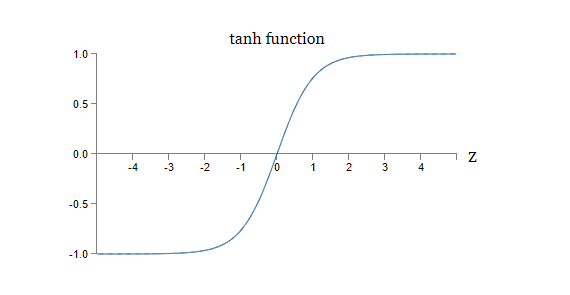tanh函数与sigmoid函数的一个不同之处在于，tanh的输出范围是 $[-1, 1]$，而不是 $[0, 1]$。这意味着如果你要使用tanh神经元，你可能需要去标准化所期望的输出。

*有一些评论认为，无论是对于tanh神经元，sigmoid神经元，还是纠正线性神经元（rectified linear neurons），这个陈述并不成立。不过，在某种程度上这样认为，并没什么问题啦！

#### 习题

• 证明公式（111）

*Efficient BackProp, by Yann LeCun, Léon Bottou, Genevieve Orr and Klaus-Robert Müller (1998)

*Understanding the difficulty of training deep feedforward networks, by Xavier Glorot and Yoshua Bengio (2010)

Sigmoid神经元的另外一个变种是rectified linear neuron（纠正线性神经元），也称作rectified linear unit（纠正线性单元）。对于一个输入为 $x$，权值向量为 $w$，bias为 $b$ 的纠正线性单元，其输出为：
$\text{max}(0, w \cdot x + b). \tag{112}$<